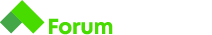# Most Frequent

Здравейте,
имам проблем със следната задача:

# Most Frequent

Write a program that finds the most frequent number in an array of N elements.

## Input

• On the first line you will receive the number N
• On the next N lines the numbers of the array will be given

## Output

• Print the most frequent number and how many time it is repeated
• Output should be `REPEATING_NUMBER (REPEATED_TIMES times)`

## Constraints

• 1 <= N <= 1024
• 0 <= each number in the array <= 10000
• There will be only one most frequent number

Кода минава с 80 точки и се чупи на два от тестовете.

``````    Scanner scanner = new Scanner(System.in);
int input = scanner.nextInt();
int[] numbers = new int[input];
int counter = 1;
int check = 0;
int result = 0;

for (int i = 0; i < numbers.length; i++) {
numbers[i] = scanner.nextInt();
}

Arrays.sort(numbers);

for (int i = 0; i < numbers.length - 1; i++) {
if (numbers[i] == numbers[i + 1]){
counter++;
}else {
if (counter > check) {
check = counter;
result = numbers[i];
}
counter = 1;
}
}

System.out.println(result + " (" + check + " times)");
``````

Test case #11: Wrong Answer [0.2 s, 31.1 MB]

0 (0 times)

Здравей,

според мен counter не ти работи, когато има само различни числа.
counter винаги е поне 1 ( винаги има поне едно число ) и не може да е 0.

Здравей,
тъй като сортирането става във възходящ ред, проблема се появява когато най-голямото число се повтаря най-често. Тогава най-дългата последователност е в края на сортирания масив. Но цикъла ще завърши без да влезе в else, тъй като всички последни са равни.
Бих пробвал да добавя още малко логика, можеби един if в тялото на първия if, какво да се случва когато `i==numbers.length-2` (т.е. достигнали сме края на цикъла) && `counter>check`. Пиши ако има нещо.

1 Like

Мерси,
получи се.

1 Like

Здравейте!Беше полезен и на мен този отговор!Само не мога да си представя (схвана) ,как така при i==numbers.length-2 разбираме ,че сме стигнали краят на масива ?Ако бихте могли да го илюстрирате по някакъв начин ще съм благодарен!

Здравей,
Тъй като броенето започва от нула, а дължината ни се дава като брой елементи (напр. 0, 1, 2, 3 има четири елемента, но последния е с индекс 3), то последния елемент е броя елементи минус едно. Но сравнявайки два съседни елемента, когато стигнем края, ние ще сравняваме предпоследния с последния. Затова казваме, че като стигнем предпоследния, сравняваме го със следващия(който се явява последен) и цикъла приключва. А предпоследния всъщност е на позиция `numbers.length-2`. Надявам се че съм отговорил на въпроса. Успех!